Structural Bioinformatics Library Template C++ / Python API for developping structural bioinformatics applications.
User Manual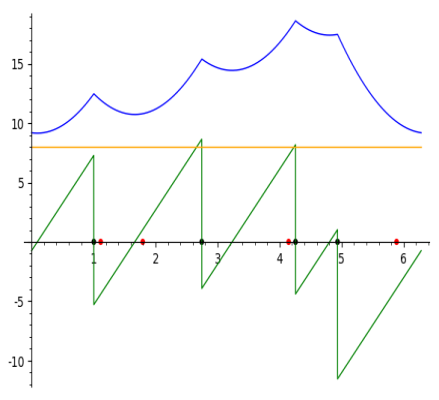# Frechet_mean_S1

Authors: F. Cazals and T. O'Donnell

# Introduction

Centers of mass and their generalization.

The celebrated center of mass of a point set in a Euclidean space is the (a) point minimizing the sum of squared Euclidean to points in this set.

Similarly, the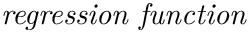mean (resp. p-mean) of a point set is a metric space is the point minimizing the sum of squared distances (resp. p-th powers of distances) to a finite point set.

This package provides a method to compute these quantities for points located on the unit circle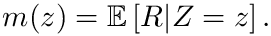, following the algorithm from  .

Definitions. Consider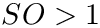angles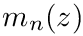. Also consider the embedding on an angle onto the unit circle, that is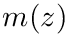.

The geodesic distance between the two points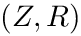and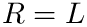on, denoted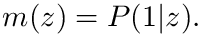, satisfies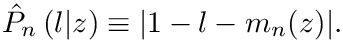This distance is also directly expressed using the angles: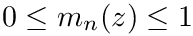For an integer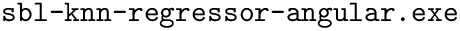, consider the function involving the p-th powers of distances to all points, that is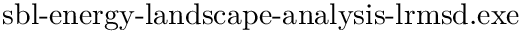Consider the minimum of this function, that is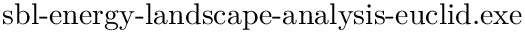The value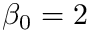obtained for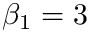, corresponds to themean. For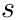, the minimum is the so-called p-mean.

Illustrations.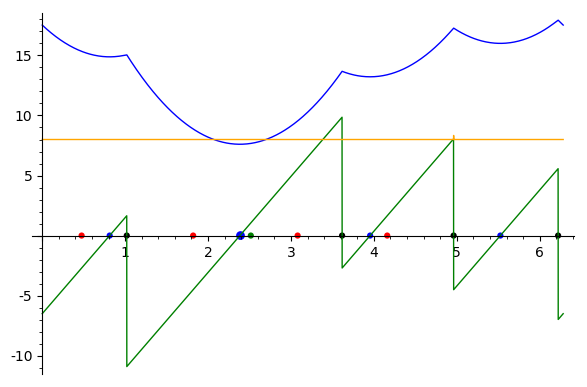Sum of square distances function Sample points are in red, their antipodal points on the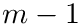circle are in black, minimas are in blue and the larger blue dot is the global minima. In blue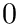, In green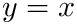and in yellow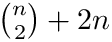.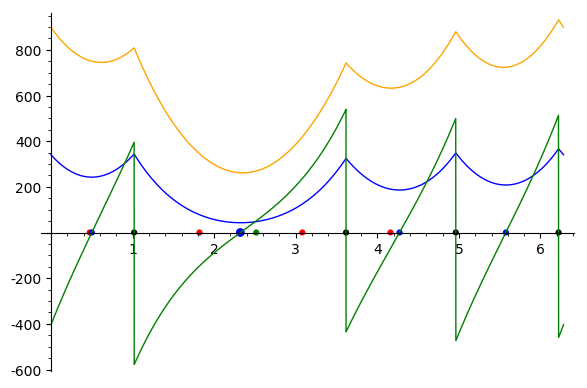Sum of distances to the power 5 function Sample points are in red, their antipodal points on thecircle are in black, minimas are in blue and the larger blue dot is the global minima. In blue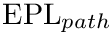, In green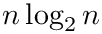and in yellow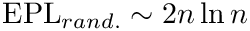.

# Algorithm

In short, the algorithm  subdivides the unit circle into interval namely circle arcs defined by data points and their antipodal values. This set of angles is denoted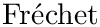. These intervals are such that there is at most one local minimum of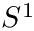on each interval. To spot these local minima efficiently, the algorithm maintains a polynomial expression of the functionand its derivative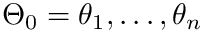. In short, the steps are:

• Construct the set• Sorting angles in• For all intervals in I:
• Identifying the intervals where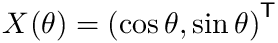vanishes
• Computing the unique root ofon such intervals
• Evaluatingat local minima, to maintain the global minimum
• Maintaining the polynomialsandat angles inAlbeit simple, the algorithm is numerically challenging since it deals with piecewise polynomials with transcendental coefficients (involving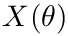).

# Implementation

Following the classical design pattern in computational geometry, this package provides a generic implementation, templated by a kernel providing number types and associated predicates and constructions.

The main class is SBL::GT::T_Frechet_mean_S1.

Two kernels are provided:

• SBL::GT::Inexact_predicates_kernel_for_frechet_mean: naive kernel using floating point representations. Therefore, no guarantee on predicates and constructions.
• SBL::GT::Lazy_exact_predicates_kernel _for_frechet_mean: kernel using a representation of angles and functions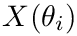using interval arithmetic. This representation guarantees exact predicates.

Note that in our case, an erroneous evaluation of predicates may yield the loss or erroneous identification of a local minimum.

# Executables

This package provides two executables:

• sbl-Frechet-mean-using-exact-predicates
• sbl-Frechet-mean-using-inexact-predicates

This corresponds to the two above mentionned kernels.

# Jupyter demo

See the following jupyter notebook:

• Jupyter notebook file
• centroid-circle
In :
import re  #regular expressions
import sys #misc system
import os
import shutil
#import shutil # python 3 only

#input filename  and output directory
odir = "results"
if not os.path.exists(odir):
os.system("mkdir results")
#choose value of p
p=4
angles_file="data/angles.txt" #file containing angles
weights_file="data/weights.txt" #file containing weights
exe_file="sbl-Frechet-mean-using-real-value-NT.exe" #App to compute minimas (sbl-Frechet-mean-using-interval-NT.exe is another option)

# run command(-r is used for specifiying radii input data -a to get all minimas)
cmd = "./%s -f %s -w %s -r -p %d -a -o %s" % (exe_file,angles_file,weights_file,p,odir)
os.system(cmd)


Out:
0
In :
# load visualisation script and instanciate using data and corresponding minimas
minimas_file="results/angles_local_minima.txt"
algo = Project()
algo.run(p)

[1.11764, 4.06681, 5.02666, 4.5149, 0.483089, 4.84806, 6.04743, 0.819754, 1.1358, 4.96215, 5.69144, 0.0488152, 5.8986, 1.04386, 6.1123, 5.30732, 0.492397, 1.57468, 2.25474, 1.01543, 1.08929, 4.58526, 0.147255, 5.77245, 0.00841053, 5.79457, 5.29714, 4.62587, 5.73067, 0.812386]
[1.11764, 4.06681, 5.02666, 4.5149, 0.483089, 4.84806, 6.04743, 0.819754, 1.1358, 4.96215, 5.69144, 0.0488152, 5.8986, 1.04386, 6.1123, 5.30732, 0.492397, 1.57468, 2.25474, 1.01543, 1.08929, 4.58526, 0.147255, 5.77245, 0.00841053, 5.79457, 5.29714, 4.62587, 5.73067, 0.812386]
[1.11764, 4.06681, 5.02666, 4.5149, 0.483089, 4.84806, 6.04743, 0.819754, 1.1358, 4.96215, 5.69144, 0.0488152, 5.8986, 1.04386, 6.1123, 5.30732, 0.492397, 1.57468, 2.25474, 1.01543, 1.08929, 4.58526, 0.147255, 5.77245, 0.00841053, 5.79457, 5.29714, 4.62587, 5.73067, 0.812386]

== Std dev angle from resultant: angle:350.956005808192 var:1.11094971447841

== Running with: num points: 8 exponent: 4
('Angle defining the Frechet mean:', 6.17846555205993, 354.000000000000)
('std dev angle from geo:', 354.000000000000, 1.11137569356359)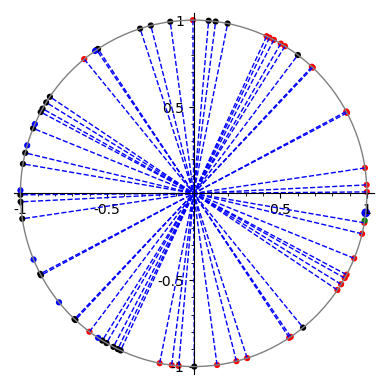here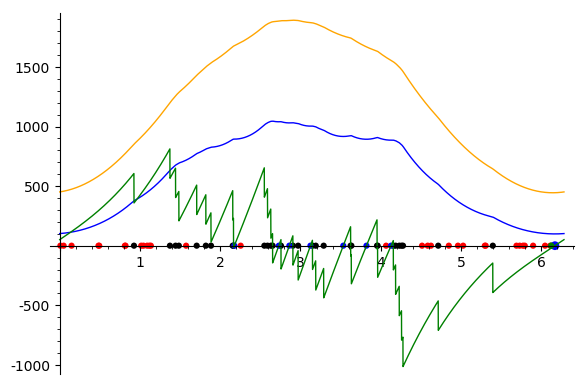done with formal expressions
[1.14926234641021, 1.40848734641021, 1.46397234641021, 1.59537234641021, 1.76351234641021, 1.85281234641021, 2.02030734641021, 2.16063734641021, 2.35778734641021, 2.56946234641021, 2.60996734641021, 2.64191734641021, 2.70499234641021, 2.83142234641021, 2.93827234641021, 3.06035526500000, 3.17020551858979, 3.23962775358979, 3.45676465358979, 3.62933565358979, 3.79398415358979, 3.95766265358979, 4.05918465358979, 4.17123765358979, 4.20816765358979, 4.24505765358979, 4.26831265358979, 4.49683265358979, 5.05630265358979, 0.0383646928204140]

In :
#change value of p
p=5
# rerun command(-r is used for specifiying radii input data)
cmd = "./%s -f %s -w %s -r -p %d -a -o %s" % (exe_file,angles_file,weights_file,p,odir)
algo.run(p)

== Std dev angle from resultant: angle:350.956005808192 var:1.11094971447841

== Running with: num points: 8 exponent: 5
('Angle defining the Frechet mean:', 6.19591884457987, 355.000000000000)
('std dev angle from geo:', 355.000000000000, 1.11206960096516)here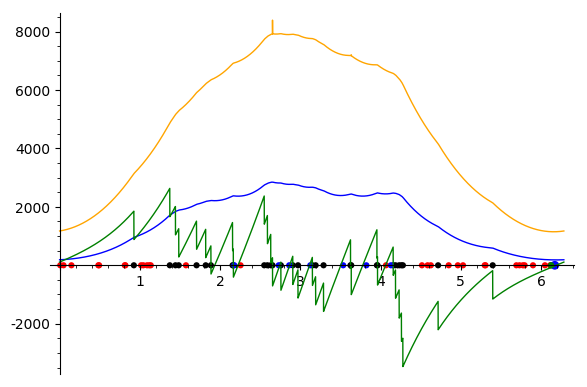done with formal expressions
[1.14926234641021, 1.40848734641021, 1.46397234641021, 1.59537234641021, 1.76351234641021, 1.85281234641021, 2.02030734641021, 2.16063734641021, 2.35778734641021, 2.56946234641021, 2.60996734641021, 2.64191734641021, 2.70499234641021, 2.83142234641021, 2.93827234641021, 3.06035526500000, 3.17020551858979, 3.23962775358979, 3.45676465358979, 3.62933565358979, 3.79398415358979, 3.95766265358979, 4.05918465358979, 4.17123765358979, 4.20816765358979, 4.24505765358979, 4.26831265358979, 4.49683265358979, 5.05630265358979, 0.0383646928204140]